Students get through the TN Board 12th Chemistry Important Questions Chapter 4 Transition and Inner Transition Elements which is useful for their exam preparation.

## TN State Board 12th Chemistry Important Questions Chapter 4 Transition and Inner Transition Elements

Question 1.
Write the electronic configuration of (a) Cr+3, (b) Cu+, (c) CO+2, (d) Mn+2, (e) Pm+3, (f) Ce+4, (g) Lu+2, (h) Th+4.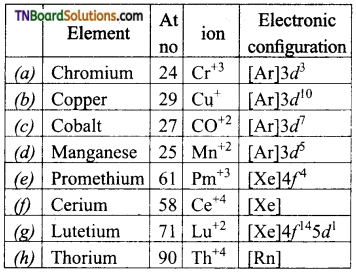Question 2.
To what extent do the electronic configuration decide the stability of oxidation states in the first series of transition elements. Illustrate your answer with an example.
In a transition, the series the oxidation state which leads to exactly half filled or completely filled ‘d’ orbitals are more stable. For example, the electronic configuration of Fe(z = 96) is [Ar] 3d6 4s2. It shows +2 and +3 oxidation states. The +3 oxidation state is more stable because it has the configuration [Ar] 3d5.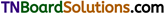Question 3.
Explain why transition elements have many irregularities in their electronic configuration.
In the transition elements, there is a little difference in the energy of (n – 1 )d and ns orbitals. The incoming electron may occupy either of these orbitals. Hence, there is irregularities in their electronic configurations.

Question 4.
Name the oxometal anoins of the first series of the transition metals in which the metal exhibits the oxidation state equal to its group number.
Cr2O7-2 (dichromate) and CrO4-2 (Chromate):
Group number of Cr=Oxidation state of Cr = 6
MnO4 (permanganate):
Group number of Mn=Oxidation state of Mn=7
Group number of V = Oxidation state of V = 5.

Question 5.
What are the stable oxidation states of the transition elements with the electronic configuration in their ground states of their atoms 3d3, 3d5, 3d8, and 3d4.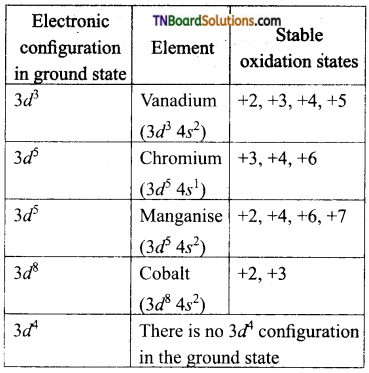Question 6.
For M+2/M and M+3/M+2 systems, the E° values for some metals are as follows:
Cr+2/Cr = -0.9V; Cr+3/Cr+2 = -0.4V
Mn+2/Mn = -1.2V; Mn+3/Mn+2 = +1.5 V
Fe+2/Fe – -0.4V; Fe+3/Fe+2 = +0.80V
Use this data to comment upon:
(i) the stability of Fe+3 in acid solution as compared to Cr+3 or Mn+3 and
(ii) the case with which iron can be oxidised as compared to a similar process for either chromium or manganese metal.
(i) Higher the reduction potential of species, greater is the tendency for its reduction to take place. Therefore, Mn+3, with the highest reduction potential would be readily reduced to Mn+2 (Mn+3 + e → Mn+2) and hence is least stable. Thus, from the values of reductions potential, it is clear that the stability of Fe+3 in acidic solution is more than Mn+3, but less than that of Cr+3.
(ii) Lower the reduction potential or higher the oxidation potential of a species, greater the case with which its oxidation will take place. Thus, the order of tendency to undergo oxidation is Fe < Cr < Mn.
Note: Oxidation and reduction potentials have the same magnitude but opposite in sign.Question 7.
How is the variability of oxidation states of transition metals different from that of non-transition metals? Illustrate with examples.
In transition elements, the successive oxidation states differ by unity. For example, manganese shows all oxidation states +2 to +7. On the other hand, non-transition metals exhibit variable oxidation states which differs by two units.eg: Pb(II) and Pb (IV); Sn(II) and Sn(IV).

Question 8.
How would you account for the following?
(i) Many of the transition elements and then compounds can act as good catalysts.
(ii) The metallic radii of the third (5d) series of transition elements are literally the same as those of the corresponding members of the second series.
(iii) There is a greater range of oxidation states among actinoids than those of lanthanoids.
(i) The catalytic activity of transition elements is attributed to the following reasons:

• Because of their variable oxidation states transition metals form unstable intermediates and provide a new path of lower activations energy for the reaction.
• In some cases, the transition metal provides a suitable large surface area with free vacancies, on which reactants are absorbed.

(ii) This is due to filling of 4/orbitals which have poor shielding effect or due to lanthanoid contraction.
(iii) This is due to comparable energies of 5f, 5d and 7s orbitals in actinoids.

Question 9.
Calculate the number of unpaired electrons in the following gaseous ions.
Mn+3, Cr+3, V+3 and Ti+3 Which one of these is the most stable in an aqueous solution?
Mn+3 = [Ar] 3d4 – 4 unpaired electrons
Cr+3 = [Ar] 3d3 – 3 unpaired electrons
V+3 = [Ar] 3d2 – 2 unpaired electrons
Ti+3 = [Ar] 3d1 – 1 unpaired electron
Cr+3 is most stable as it is half-filled t2g level.

Question 10.
The electronic configuration of chromium and copper are [Ar] 3d5 4s1 and [Ar] 3d10 4s1 respectively instead of [Ar] 3d4 4s2 and [Ar] 3d9 3s2. Explain.
The electronic configuration [Ar] 3d5 4s1 and [Ar] 3d10 4s1 is more stable than the other because of the extra stability of half filled and completely filled ‘d’ orbitals. This extra stability is due to the symmetrical distribution of electron density.Question 11.
The melting and boiling points of Zn, Cd and Hg are low why? (or) Why Zn, Cd and Hg are soft and have low melting points?
In Zn, Cd and Hg, all the electrons in the ‘d’ orbitals are paired. Hence metallic bonding present in than are weak. Hence, the attraction between the constituent atoms are weak, i.e., they have low melting and boiling points.

Question 12.
Account for the trend in melting points of 3d series.
The melting point first increases as the number of unpaired ‘d’ electrons available for metallic bonding increases, reaches a maximum and then decreases, as the ‘d’ electrons pair up and becomes unavailable for metallic bonding. The maximum melting point is in the middle, where the transition metal has d5 configuration. This results in the formation of strong metallic bonding, which results in the maximum melting point in the middle of the series.

Question 13.
Explain why manganese has a lower melting point than chromium.
The electronic configuration of chromium is [Ar] 3d5 4s1 and that of manganese is [Ar] 3d5 4s2 i.e., in manganese-all the ‘d’ orbitals are singly filled and the 4s orbital is completely filled. As the ‘d’ electrons are more tightly held by the nucleus, the electrons are not available for bonding resulting in weaker metallic bonding in manganese compared to chromium.

Question 14.
The second and third members of each group of transition elements have similar atomic radii- Explain.
This is due to lanthanide contraction by members of 4f series, which occupy a position between lanthanum (at. no. 57) a first member of 3rd transition series and hafnium (Z = 72), the second member of third transition series.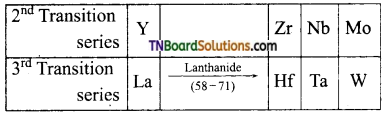The pairs of elements Zr – Hf, Nb – Ta, Mo – Wo, possess nearby atomic radii and almost have the same properties.

Question 15.
The atomic radii of the elements in a transition series do not vary much while they do vary in case of ‘s’ and ‘p’ block elements explain.
In transition elements two effects are operating, viz nuclear charge effect and screening effect which oppose each other. Due to an increase in nuclear charge from member to member in a transition series the atomic radii tend to decrease. At the same time, the addition of extra electrons in (n – 1 )d orbital provides the screening effect. As the number of ‘d’ electrons increases, the screening effect increases and this tends to increase the size. Due to these opposing tendencies, there is a small change in the atomic radii in a transition series.
In ‘s’ and ‘p’ block elements, the extra electron is added to the same ‘s’ or ‘p’ subshell which does not exert a screening effect and hence, the atomic radii considerably in a period due to an increase in nuclear charge.Question 16.
How would you account for the following?
(i) The atomic radius of metals of the third (5d) series of elements are virtually the same as those of corresponding members of the second (4d) series.
(ii) Chromium is a typical hard metal while mercury is a liquid.
(i) Due to lanthanoid contraction.
(ii) Chromium has five unpaired electrons in the ‘d’ subshell (3d5 5s1). Hence metallic bonds are very strong. In mercury, all the ‘d’ orbitals are fully filled (3d10 4s2). Hence, metallic bonding is weak and thus it exists as a liquid.

Question 17.
How would you account for the irregular variation of ionisation enthalpies (first and second) in the first series of transition metals?
Reason for irregular variation of first IE: Generally moving from left to right in a period IE increase because of increase in nuclear charge. The irregular trend is observed, when an electron is removed, the relative energies of 3d and 4s orbitals are altered. Thus there is a reorganisation of energy accompanying ionisation. This results in the release of exchange energy which increases as the number of electrons increases in the ‘d’ configuration and also from the transfer of ‘s’ electrons into ‘d’ orbitals. Chromium has the first low IE because of the loss of one electron gives a stable configuration (d5). Zn has high IE because the electron has to be removed from 4s orbital of the stable configuration (3d10 4s2)
Reason for irregular variation of second IE: After the loss of one electron, the removal of the second electron is difficult. Hence, second IE is much higher and in a general increase from left to right. Cr and Cu show much higher values because the second electron has to be removed from the stable configuration. Cr+(3d5) and Cu+(3d10).

Question 18.
Compare the stabilities of Ni+4 and Pt+4 from their ionisation enthalpy values.

 IE Ni Pt I 737 864 II 1753 1791 III 3395 2800 IV 5297 4150

The smaller the magnitude of the ionisation energy, the more stable is the compound in a particular oxidation state. Nickel (II) compounds are more stable than Pt(II) compounds while Pt(IV) compounds are more stable than Ni(IV) compounds. The sum of the first two and first four IE of nickel and platinum are given below.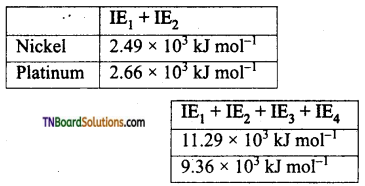Since the first two ionisation energies is less for nickel than platinum, Ni(II) compounds are more stable than platinum.
On the other hand, Pt(IV) compounds are more stable than Ni(IV) compounds as the seem of the first four IE values are less than for Pt the Ni.

Question 19.
The silver atom has completely ‘d’ orbitals (4d10) in its ground state. How can you say that it is a transition element?
The electronic configuration of Ag (z = 47) to 4d10 5s1. In addition to +1, it shows an oxidation state of +2 (eg: Ag2O, and F2). i.e., d9 configuration i.e., the ‘d’ orbital is incompletely filled. Hence, it is a transition element.Question 20.
Which of the 3d transition metals exhibit the largest number of oxidation states? and why?
Manganese (z = 25) shows a maximum number of oxidation states. This is because its electronic configuration is 3d5 4s2. As 3d and 4s are close in energy, it has a maximum number of electrons to lose or share (all the 3d electrons are unpaired) Hence, it shows oxidation state +2 to +7.

Question 21.
Why is the highest oxidation state of a metal exhibited in its oxide or fluoride only?
Oxygen and fluorine have small sizes and high electronegativity. Hence they can oxidise the metal to its highest oxidation state.

Question 22.
What may be the stable oxidation state of the transition element with the following ‘d’ electronic configuration in the ground state of their atoms? 3d3, 3d5, 3d8 and 3d4.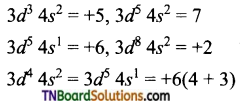The maximum oxidation state corresponds to the sum of the ‘5’ and ‘cf electrons in Upto Mn. After that there is an abrupt decrease in the stability of the higher oxidation state.

Question 23.
Why are Mn+2 compounds are more stable than Fe+2 compounds towards oxidation to +3 state?
The electronic configuration of Mn is 3d5. Which is half-filled and hence stable. So, the third ionisation enthalpy is very high, i.e., 3rd electron cannot be removed easily. In the case of Fe+2, (3d6), it can lose one more electron to attain the stable (d5) configuration.

Question 24.
Explain briefly how +2 oxidation state becomes more and more stable in the first half of first-row transition elements with increasing atomic number.
The sum of IE1, and IE2 increases. Thus, the standard reduction potential becomes less and less negative. Therefore, the tendency to form M+2 ion decreases. The greater stability of +2 state, say for Mn, is due to half-filled (d5) configuration and that for Zn is due to completely filled ‘d’ orbitals. That for nickel is due to the highest negative enthalpy of hydration.

Question 25.
To what extent do the electronic configuration decide the stability of oxidation states in the first series of transition elements? Illustrate your answer with an example.
The stability of the oxidation state in the first transition elements are related to their electronic configuration.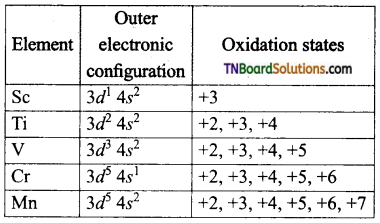The first five elements of the first transition series up to Mn in which 3d subshell is not more than half-filled, the minimum oxidation state is given by the number of electrons in the outer s – subshell and the maximum oxidation state is given by the sum of outer ‘s’ and ‘d’ electrons.

Question 26.
Explain why E°(Mn+3/Mn+2) couple is more positive than for E°(Fe+3/Fe+2) (At.no.of Mn = 25; Fe = 26).
The electronic configuration of Mn+2 is Mn+2 = [Ar] 3d5; Mn+3 is 3d4 and that of Fe+2 = [Ar] 3d6; Fe+3 is [Ar] 3d5.
Thus Mn+2 has a more stable configuration than Mn+3 while Fe+3 has a more stable configuration than Fe+2. Consequently, large third ionisation enthalpy is required to change Mn+2 to Mn+3. As E° values is the sum of the enthalpy of atomisation, ionisation enthalpy and hydration enthalpy, therefore E° for Mn+3/ Mn+2 couple is more positive than Fe+3/Fe+2.
Note: The large positive E° for Mn+3/ Mn+2 means that Mn+3 can be easily reduced to Mn+2 (Mn+3 + e → Mn+2) i.e., Mn+3 is less stable, i.e., Fe+3 can be reduced to Fe+2 (Fe+3 + e → Fe+2 but ion easily. Thus, Fe+3 is more stable than Mn+3.Question 27.
The E°values in respect of the electrodes of chromium (z = 24), manganese (z = 25) and iron (z = 26) are: Cr+3/Cr+2 = – 0.4V, Mn+3/Mn+2 = 1.5V; Fe+3/Fe+2 – 0.80V. On the basis of the above information compare the feasibilities of further oxidation of their +2 oxidation states.
Cr+3 + e -> Cr+2: E° value is -0.4V. The negative value means Cr+3 is more stable or Cr+2 is less stable. Mn+3 + e → Mn+2: E° value is 1.5V. Fe+3 + e → Fe+2: E° value is 0.80V. Greater positive value for Mn+3/Mn+2 than that for Fe+3/Fe+2 shows that Mn+2 is more stable than Fe+2. Hence, the stability of +2 oxidation state is in the order Cr+2 < Fe+2 < Mn+2 or oxidation of their +2 state is in the order Cr+2 > Fe+2 > Mn+2.

Question 28.
The E°(M+2/M) value for copper is positive 0.34V. Explain why?
E°(M+2/M) for any metal is related to the sum of the enthalpy change taking place in the following steps.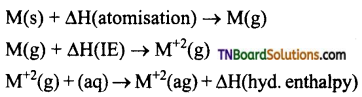Copper has a high enthalpy of atomisation and low enthalpy of hydration. Thus, high energy required to transform Cu(s) to Cu+2(aq) is not balanced by its hydration enthalpy Hence E°(Cu+2/Cu) is positive.

Question 29.
Which is a stronger reducing agent Cr+2 or Fe+2 and why?
E°(Cr+3/Cr+2) = -0.41V and E°(Fe+3/Fe+2) is 0.77V.
In general, for the reduction reaction Mn+ + ne → M,
Larger the value of standard reduction potential, greater the extent to which Mn+ is reduced to M. i.e., Mn+ acts as an oxidising agent. Compare
Cr+3 + e → Cr+2 E° = -0.41V
Fe+3 + e → Fe+2 E° = 0.77V.
The negative value indicates that Cr+2 is readily oxidised to Cr+3 i.e., it is a stronger reducing agent than Fe+2.Question 30.
Explain the terms paramagnetism and diamagnetism with suitable examples.

1. A paramagnetic substance is one that is weakly attracted into a magnetic field and a diamagnetic substance is one that is repelled by a magnetic field.
2. The paramagnetic behaviour arises due to the presence of one or more unpaired electrons, while a diamagnetic substance is due to the presence of paired electrons.
3. For example Sc+3 has no unpaired electron and hence diamagnetic whereas Ti+3 has one unpaired electron and hence paramagnetic.

Question 31.
Calculate the magnetic moment of the following ions.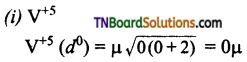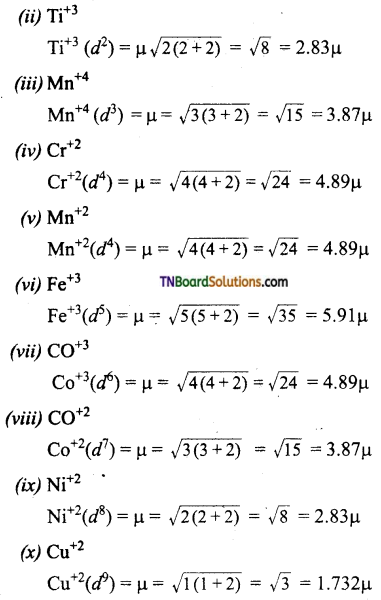Question 32.
Explain how transition metals and their compounds act as catalysts.
Transition metal has energetically available ‘d’ orbitals that can accept electrons from reactant molecule or metal can form a bond with reactant molecule using its ‘d’ electrons. For example, in the catalytic hydrogenation of an alkene, the alkene bonds to an active site by using its π electrons with an empty ‘d’ orbital of the catalyst.
The σ bond in the hydrogen molecule breaks and each hydrogen atom forms a bond with a ‘d’ electron on an atom in the catalyst. The two hydrogen atoms then bond with the partially broken π -bond in the alkene to form an alkane.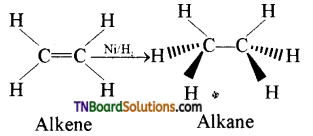Question 33.
Give examples wherein transition metal compounds act as catalysts in various industrial processes.

1. In the manufacture of sulphuric acid from sulphur trioxide, vanadium pentoxide is used as a catalyst.
2. Hydroformylation of olefins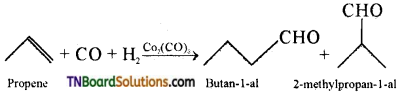3. Preparation of acetic acid from acetaldehyde.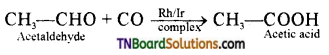4. Zeigler – Natta catalyst
A mixture of TiCl4 and trialkyl aluminium is used for polymerization.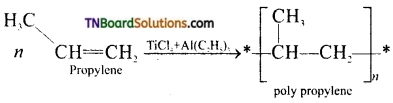Question 34.
What is an alloy? How are they formed?
An alloy is a mixture of two or more metals. They are formed when molten metals are mixed together and allowed to crystallise, eg: ferrous alloys, gold – copper alloy, chrome alloys etc.Question 35.
Explain why transition metals have a tendency to form alloys.
For the formation of alloy, the following conditions have to be met.

1. Both the solute and the solvent must have the same crystal structure.
2. Their valence and their electronegativity difference must be close to zero. Transition metals satisfy these conditions. Their atomic sizes are similar and one metal atom can be easily replaced by another metal atom from its crystal lattice to form an alloy.

Question 36.
What are interstitial compounds? Give examples.
An interstitial compound or alloy is a compound that is formed when small atoms like hydrogen, boron, carbon or nitrogen are trapped in the interstitial holes in a metal lattice. They are usually non-stoichiometric compounds. Transition metals form a number of interstitial compounds such as TiC, ZrH1.92, Mn4N etc., The elements that occupy the metal lattice provide them new properties.

Question 37.
Mention the properties of interstitial compounds.

1. They are hard and show electrical and thermal conductivity.
2. They have high melting points higher than those of pure metals.
3. Transition metal hydrides are used as powerful reducing agents.
4. Metallic carbides are chemically inert.

Question 38.
What are complexes or coordination compounds? Give a brief account of complexes formed by transition metals.
Complexes or coordination compounds are these in which a transition metal ion accept a pair of electrons, from a fixed number of compounds in which the central atom can denote a pair of electrons and form coordinate covalent bonds.
eg: [Fe(CN)6]4-. In this complex, Fe+2 ion accepts lone pair of electrons from the cyanide ion and form 6 coordinate covalent bonds. Transition metal ions form complexes due to their small size and high charge and have vacant ‘d’ orbitals to accept electron pairs denoted by other groups.

Question 39.
Give a brief account of oxides formed by transition metals.

1. The metals of the first transition series form oxides with oxygen at high temperatures.
2. The oxidation state of the metal in the oxides vary from +1 to +7.
3. The highest oxidation state in the oxides of any transition metal is equal to its group number eg: +7 in Mn2O7. Beyond group 7. No higher oxides of iron above Fe2O3 are known.
4. All the metals except scandium form oxides of the formula MO which are ionic in nature. As the oxidation number of the metal increases, ionic character decreases, eg: Mn2O7 is covalent even CrO3, V2O5 are covalant and have low melting point.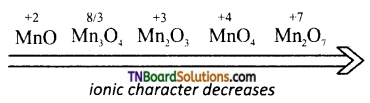5. In general, the oxides in the lower oxidation states of the metals are basic and in their higher oxidation states they are acidic. Whereas in the intermediate oxidation states they are amphoteric.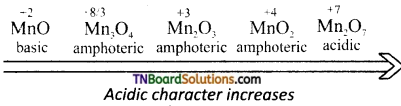Question 40.
Classify the following oxides as acidic, basic and amphoteric
(a) Mn2O7, (b) CrO3, (c) Cr2O3, (d) CrO.
(a) Mn2O7 → acidic
(b) CrO3 → acidic
(c) Cr2O3 → amphoteric
(d) CrO → basic.

Question 41.
Give example and suggest the reason for the following:
(i) The lowest oxide of transition metal is basic, the highest is acidic.
(ii) A transition metal exhibits higher oxidation states in oxides and fluorides.
(iii) The highest oxidation state is exhibited in oxo anion of a metal.
(i) The lower oxide of a transition metal is basic because the metal atom has a low oxidation state whereas the highest oxide is acidic due to a higher oxidation state for example, MnO is basic while Mn2O7 is acidic.
(ii) A transition metal exhibits a higher oxidation state in oxides and fluorides because oxygen and fluorine are highly electro negative elements, small in size (and strongest oxidising agents). For example, osmium shows an oxidation state of +6 in O5F6 and vanadium shows an oxidation state of +5 in V2O5.
(iii) Oxo metal anion have the highest oxidations state, eg: Cr in Cr2O7-2, has an oxidation state of +6, whereas Mn in MnO4 ion has an oxidation state of +7. This again due to the combination of the metal with oxygen which is a highly electronegative and oxidising element.

Question 42.
Indicate the steps involved in the preparation of (i) K2Cr2O7 from chromite (ii) KMnO4 from pyrolusite
(i) K2Cr2O7 from chromite are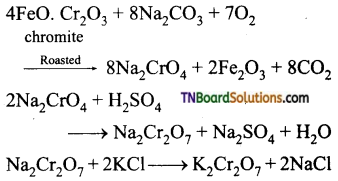K2Cr2O7 is separated by fractional crystallisation.
(ii) KMnO4 from pyrolusite are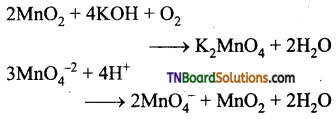KMnO4 is prepared from pyrolusite. MnO2 with KOH in the presence of an oxidising agent like KNO3. This produces dark brown potassium manganate which disproportionates in a neutral or acidic solution to give purple permanganets.

Question 43.
Give chemical oxidation and electrolytic oxidation of MnO4-2 to MnO4.
Chemical oxidation: In this method potassium manganate is treated with ozone (O3) or chlorine to get potassium permanganate.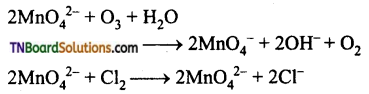Electrolytic oxidation: In this method aqueous solution of potassium manganate is electrolyzed in the presence of little alkali.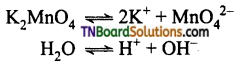Manganate ions are converted into permanganate ions at anode.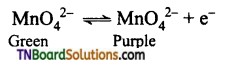H2 is liberated at the cathode.
2H+ + 2e → H2 ↑
The purple coloured solution is concentrated by evaporation and forms crystals of potassium permanganate on cooling.Question 44.
What happens when potassium dichromate is heated? Give equation.
It decomposes to give green Cr2O3 and oxygen.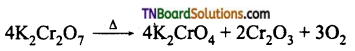Question 45.
Discuss the action of alkali on potassium dichromate.
When an alkali is added to an orange-red solution of potassium dichromate, a yellow solution results due to the formation of chromate.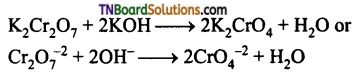Question 46.
What is the effect of pH on a solution of potassium dichromate?
In aqueous solution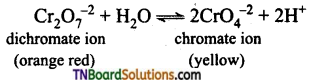In acidic medium (i.e., decreasing pH), the equilibrium shifts backwards and the colour is orange-red. In a basic medium, (i.e., increasing pH) the equilibrium will shift forward and the solution is yellow.

Question 47.
Draw the structure of the dichromate ion.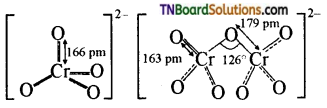Question 48.
Write the ionic equation to show that K2Cr2O7 is an oxidising agent in an acid medium.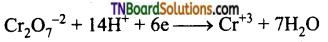Question 49.
Complete and balance the following equation: (all in acid medium)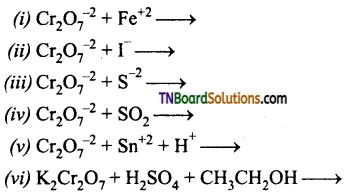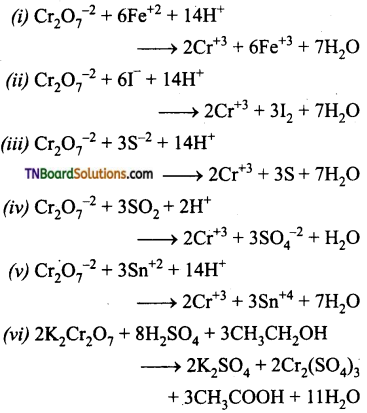Question 50.
Describe the chromyl chloride test.
When potassium dichromate is heated with any chloride salt in the presence of Conc. H2SO4, orange-red vapours of chromyl chloride (CrO2Cl2) is evolved. This reaction is used to confirm the presence of chloride ions in inorganic qualitative analysis.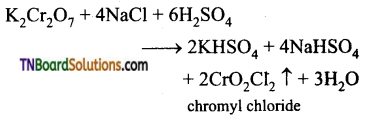The chromyl chloride vapours are dissolved in sodium hydroxide solution and then acidified with acetic acid and treated with lead acetate. A yellow precipitate of lead chromate is obtained.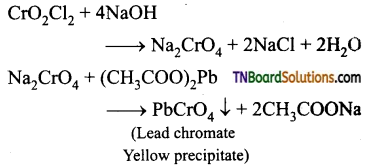Question 51.
Mention the uses of potassium dichromate.

1. It is used as a strong oxidizing agent.
2. It is used in dyeing and printing.
3. It used in leather tanneries for chrome tanning.
4. It is used in quantitative analysis for the estimation of iron compounds and iodides.

Question 52.
Draw the structure of MnO4 ion.
Permanganate ion has tetrahedral geometry in which the central Mn7+ is sp3 hybridised.Question 53.
What happens when:
(i) potassium permanganate is heated.
(ii) is heated with cold conc. H2SO4
(iii) is heated with hot conc. H2SO4?
(i) It decomposes to form potassium
manganate and manganese dioxide.
2KMnO4 → 2K2MnO4 + MnO2 + O2
(ii) It decomposes to form manganese heptoxide which subsequently decomposes explosively.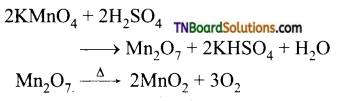(iii) It gives manganous sulphate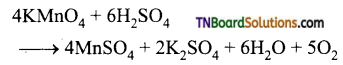Question 54.
How does potassium permanganate act as an oxidising agent in the neutral medium? Explain with examples.
In neutral medium, KMnO4 is reduced to MnO2.
MnO4 + 2H2O + 3e → MnO2 + 4OH
(i) It oxidises H2S to sulphur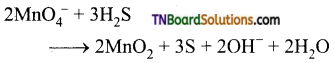(ii) It oxidises thiosulphate into sulphate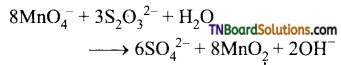Question 55.
Write an ionic equation or the half-reaction for the oxidising action of KMnO4 in an alkaline medium.
MnO4 + 2H2O + 3e → MnO2 + 4OHQuestion 56.
What is Bayer’s reagent? Mention its use.
Cold dilute alkaline KMnO4 is known as Bayer’s reagent. It is used to oxidise alkenes into diols. For example, ethylene can be converted into ethylene glycol and this reaction is used as a test for unsaturation.

Question 57.
What is the action of acidified KMnO4 on
(i) ferrous salts,
(ii) Potassium iodide,
(iii) Oxalic acid
(iv) Sulphide ion,
(v) nitrite ion,
(vi) Sulphite ion.
(i) It oxidises ferrous salts to ferric salts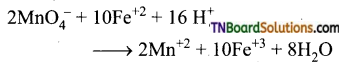(ii) It oxidises iodide ion to iodine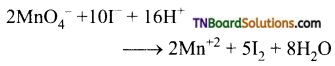(iii) It oxidises oxalic acid to carbon dioxide.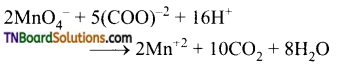(iv) It oxidises sulphide ion to sulphur.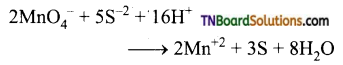(v) It oxides nitrite ion to nitrates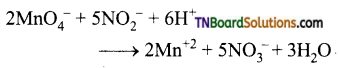(vi) It oxidises sulphite ion to sulphates.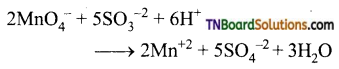Question 58.
Mention the uses of potassium permanganate.

1. It is used as a strong oxidizing agent.
2. It is used for the treatment of various skin infections and fungal infections of the foot.
3. It is used in water treatment industries to remove iron and hydrogen sulphide from well water.
4. It is used as a Bayer’s reagent for detecting unsaturation in an organic compound.
5. It is used in quantitative analysis for the estimation of ferrous salts, oxalates, hydrogen peroxide and iodides.

Question 59.
Find the equivalent weight of KMnO4 is an acidic, basic and neutral medium.
The ionic equation is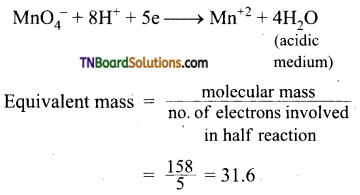In basic medium: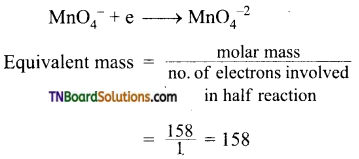In neutral medium: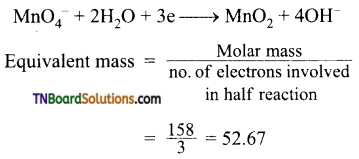Question 60.
What are inner transition elements?
Decide which of the given atomic numbers are the numbers of inner transition elements: 29, 59, 74, 95, 102, 104.
The ‘f’ block elements i.e., in which the last electron enters the ‘f’ sub shell are called inner transition elements. These include lanthanides (58 – 71) and actinides (90 – 103). Thus, the elements with atomic numbers 59,95 and 102 are inner transition elements.

Question 61.
The chemistry of actinoid elements is not so smooth as that of lanthanoids justify then statement by giving some examples from the oxidation state of these elements.
Lanthanoids show a limited number of oxidation states viz +2, +3, +4 out of which +3 is most common. This is because of the energy gap between 4f and 5d sub shells. The dominant oxidation state of actinides is also +3 but they show a number of oxidation states also eg: uranium (Z = 92) and polonium (Z = 92) show +3, +4 and +5 and +6, neptunium (Z = 94) shows +3, +4, +5 and +7 etc., This is due to small energy diffidence between 5f, 6d and7s subshells.

Question 62.
What is the last element in the series f actinoids? Comment on the possible oxidation state of the element.
Last actinoid: Lawrencium (Z = 103).
Electronic configuration; [Rn]86 5f14 6d1 7s2. possible oxidation state = +3.

Question 63.
Name of the members of the lanthanoid series which exhibit +4 oxidation state. Try to correlate this type of behaviour with the electronic configuration of these elements.
+4: Ce(Z=58), Pr(Z=59), Nd(Z=60), Tb(Z=65), Dy(Z = 66)
+2: Nd(Z = 60); Sm(Z = 62); Eu(Z = 63), Tm(Z = 69); Yb(Z = 70)
+2 Oxidation state is exhibited when lanthanoid has the configuration 5d0 6s2. So that 2 electrons one easily lost. +4 state is exhibited when the electronic configuration left to close to 4f0 (eg: 4f0, 4f1, 4f2) or close to 4f7 (eg: 4f7 or 4f8)

Question 64.
Write the electronic configuration of the elements with atomic numbers 61, 91, 101 and 109.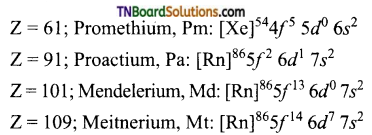Question 65.
Compare the chemistry of actinoids with that of lanthanoids with special reference to
(i) electronic configuration
(ii) Oxidation state
(iv) Chemical reactivity
(i) The general electronic configuration of lanthanoids is [Xe]54 4f1-14 5d0-1 6s2 where as that of actinoids in [Rn]86 5f1-14 6d0-1 7s2. Thus lanthanoids belong to 4f series whereas actinoids belong to 5f series.
(ii) Lanthanoids show limited oxidation states (+2, +3, +4) out of which +3 is most common. This is because of the large energy gap between 4f and 5d subshells. On the other hand, actinoids show a large number of oxidation states because of a small energy gap between 5f, 6d and 7s subshells.
(iii) Both show a decrease in the size of their atoms or ions in the +3 oxidation state. In lanthanoids, this decrease is called lanthanoid contraction, whereas in actinoids it is called actinoid contraction. However, the contraction is greater from element to element in actinoids due to poor shielding of 5f electrons than that by 4f electrons in lanthanoids.
(iv) Chemical reactivity of lanthanoids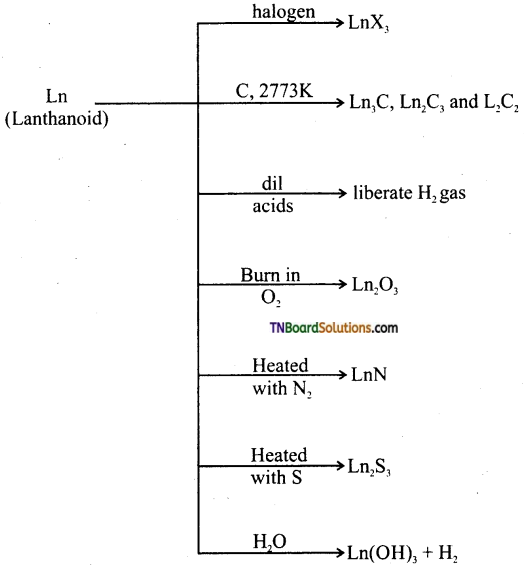The chemical behaviour of actinoids:

1. They react with boiling water to give a mixture of hydride and oxide.
2. They combine with most of the non-metals at high temperatures.
3. All these metals are attacked by hydro chloric acid, but the effect of nitric acid is very small due to the formation of a protective oxide layer on their surface.
4. Alkalies have no action on actinoids.Question 66.
The +3 oxidation state of lanthanium (Z = 57), gadoliniun (Z = 64) and lutetium (Z = 71) are especially stable. Why?
This is because that is the +3 oxidation state, they have empty, half-filled and completely filled 4f sub shell respectively.

Question 67.
The outer electronic configuration of two members of the lanthanoid are as follows:
(i) 4f1 5d1 6s2 and (ii) 4f7 5d0 6s2
What are their atomic numbers predict the oxidation state exhibited by these elements in their compounds?
Complete Electronic configuration of the (i) lanthanoid:
[Xe]54 4f1 5d1 6s2: Atomic number = 58
The element is cerium.
Complete Electronic configuration of the (ii) lanthanoid:
[Xe]54 4f7 5d0 6s2: Atomic number = 68.
The element is Europium (Eu).
Oxidation state of 1st lanthanoid +2(4f2) +3(4f1) and +4(4f0)
Oxidation state of the 2nd lanthanoid:
+2(4f7) and +3(4f6)

Question 68.
Give an explanation for each of the following observations.
(i) The gradual decrease in size (actinoid contraction) from element to element is greater among actinides than that among lanthanides.
(ii) The actinoids exhibit a greater range of oxidation states than the lanthanoids.
(iii) Zv and Hf have identical sizes.
(iv) Ionisation enthalpies of Ce, Pr and Nd are higher than Th, Pa and Cl. Why?
(v) Ce+4 is used as an oxidising agent in volumetric analysis.
(i) This is due to poor shielding by 5f electrons from element to element in actinoids than by 4f electrons in lanthanoid series.
(ii)This is because there is less energy difference between 5f and 6d orbitals belonging to actinoids than the energy difference between 4f and 5d Orbitals in the case of lanthanoids.
(iii) This is due to lanthanoid contraction.
(iv) It is because at the beginning, where 5f orbitals begin to be occupied they will penetrate less into the inner core of electrons. The 5felectron will therefore be more effectively shielded from the nuclear charge than 4f electrons of the corresponding lanthanoids. Therefore the outer electrons are less firmly held and they are available for bonding in the actinoids.
(v) Ce+4 has a tendency to attain +3 oxidation state and so it is used as an oxidising agent in volumetric analysis.

Question 69.
Account for the following:
(i) Europium (II) is more stable than cerium(II)
(ii) Actinoid ions are generally coloured.
(i) Europium has stable configuration i.e., [Xe] 4f7 5d0 6s0.
(ii) Unpaired electrons are present in their ions which undergo f-f transition.Question 70.
On the basis of lanthanoid contraction, explain the following:
(i) Nature of bonding in La2O3 and Lu2O3.
(ii) Trends in the stability of oxosaltes of lanthanoids from La to Lu.
(iii) Stability of complexes of lanthanides.
(iv) Radii of 4d and 5d block elements.
(v) Trends in the acidic character of lanthanoid oxides.
(i) As the size decreases, the covalent character increases. Therefore La2O3 is more ionic and Lu2O3 is more covalent.
(ii) As the size decreases from La to Lu, the stability of oxo salts also decreases.
(iii) Stability of the complexes increases as the size of the lanthanoid decreases.
(iv) Radii of 4d and 5d block elements are will be almost the same.
(v) Acidic character of the oxides increases from La to Lu.

Question 71.
What is meant by the term Lanthanoid contraction? What is it due to and what consequences does it have on the chemistry of lanthanides in the periodic table.
Lanthanide contraction: The steady decrease in atomic and ionic radii with an increase in atomic number is known as lanthanide contraction.
Causes of lanthanide contraction: As we move along the lanthanoid series, for every additional proton in the nucleus, the corresponding electron goes into 4f sub shell, there is poor shielding of one electron by another in the sub shell due to the shapes of these orbitals. This imperfect shielding is not able to counterbalance the effect on the increased nuclear charge. Thus, the net result is a decrease in size, with an increase in atomic number.
Consequences:

1. 5d series elements have nearly the same radii as that of the 4d series.
2. The basic strength of hydroxides decrease from La(OH)3 to Lu(OH)3

1. The correct order of decreasing second ionisation enthalpy of Ti(22), V(23), Cr(24) and Mn(25) is:
(a) Cr > Mn > V > Ti
(b) V > Mn > Cr > Ti
(c) Mn > Cr > Ti > V
(d) Ti > V > Cr > Mn
(a) Cr > Mn > V > Ti
Hint: Electronic configuration of the given elements are
Ti = [Ar] 3d2 4s2; V = [Ar] 3d3 4s2 Cr = [Ar] 3d5 4s1; Mn = [Ar] 3d5 4s2
Their effective nuclear charge increases from Ti to Mn. Hence their first IE increases in the same order, i.e., Mn > Cr > V > Ti. However after the removal of first electron, chromium attains the stable configuration [3d5] and hence, it has very high second IE. For the remaining elements the trend remains the same. Thus, the second IE will be in the order.
Cr > Mn > V >Ti2. The electronic configuration of Cu(II) is 3d9 whereas that of Cu(I) is 3d10. Which of the following is correct?
(a) Cu(II) is more stable.
(b) Cu(II) is less stable.
(c) Cu(I) and Cu(II) are equally stable.
(d) Stability of Cu(I) and Cu(II) salts depends on the nature of copper salts.
(a) Cu(II) is more stable.
Hint: Though Cu(I) has 3d10 configurations Cu(II) is more stable. This is due to greater effective nuclear charge of Cu(II). i.e., to hold 17 electrons instead of 18 electrons is Cu(I).

3. Although zirconium belongs to 4d transition series and Hafnium belongs to 5d transition series even their they have similar physical and chemical properties because:
(a) both belong to ‘cf block
(b) both have same number of electrons
(c) both have similar atomic radius
(d) both belong to the same group of the periodic table.
(c) both have similar atomic radius

4. Why HCl is not used to make the medium acidic in oxidation reactions of KMnO4 in acidic medium?
(a) Both HCl and KMnO4 act as oxidising agents.
(b) KMnO4 oxidises HCl, to Cl2, which is also an oxidising agent.
(c) KMnO4 is a weaker oxidising agent than HCl.
(d) KMnO4 acts as a reducing agent in the presence of HCl.
(b) KMnO4 oxidises HCl, to Cl2, which is also an oxidising agent.

5. KMnO4 acts as an oxidising agent in acidic medium. The number of moles of KMnO4 that will be needed to react one mole of sulphide ions in acidic solution is:
(a) $$\frac{2}{5}$$
(b) $$\frac{3}{5}$$
(c) $$\frac{4}{5}$$
(d) $$\frac{1}{5}$$
(a) $$\frac{2}{5}$$
Hint: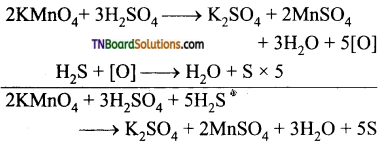2 mol of KMnO4 ≡ 5 mol H2S
$$\frac{2}{5}$$ mol of KMnO4 ≡ 1 mol of H2S

6. Which one of the following ions is the most stable in aqueous solution:
Note: Atomic No: Ti = 22, V = 23, Cr – 24, Mn = 25.
(a) Mn+2
(b) Cr+3
(c) V+3
(d) Ti+3
(b) Cr+3
Hint: Out of the given species, Cr+3 has the highest negative reduction potential. Hence, it cannot be reduced to Cr+2 and therefore is the most stable in aqueous solution.
Alternatively, Mn+3 = [Ar] 3d4, Cr+3 = [Ar] 3d3, V+3 = [Ar] 3d2, Ti+2 = [Ar] 3d1. In Cr+3, all the three electrons enter the lowest energy 3d1(t2g ) orbitals. The lowering of the energy is maximum and hence the stability is maximum.7. In acidic medium MnO4-2:
(a) disproportionates to MnO2 and MnO4
(b) is oxidised to MnO4
(c) reduced to MnO2
(d) is reduced to Mn+2
(a) disproportionates to MnO2 and MnO4
Hint: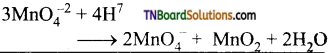8. Consider the following statements:
(i) La(OH)3 is the least basic among hydroxides of lanthanides
(ii) Zn+4 and Hf+4 possess almost same ionic radii
(iii) Ce+4 can act as oxidising agent. Which of the above is/are true?
(a) All
(b) (ii) and (iii)
(c) (ii) only
(d) (i) and (iii)
(b) (ii) and (iii)
Hint: La(OH)5, is most basic, i.e., (i) is wrong.
(ii) is correct due to lanthanide contraction.
(iii) is correct because Ce+4 tends to change to stable Ce+3.

9. Large number of oxidation states are exhibited by actinoids than these by the lanthanoids, the main reason being:
(a) more energy difference between 5f and 6d than between 4f and 5d orbitals.
(b) more reactive nature of the actinoids than the lanthanoids.
(c) 4f orbitals more diffused than 5f orbitals.
(d) less energy difference between 5f and 6d than between 4f and 5d orbitals.
(d) less energy difference between 5f and 6d than between 4f and 5d orbitals.

10. Irregular trend in the standard reduction potential value of the first row transition elements is due to:
(a) regular variation of first and second row enthalpies.
(b) irregular variation of sublimation enthalpies.
(c) regular variation of sublimation enthalpies.
(d) increase in number of unpaired electrons.
(b) irregular variation of sublimation enthalpies.11. Match the property in column I with the metals in column II.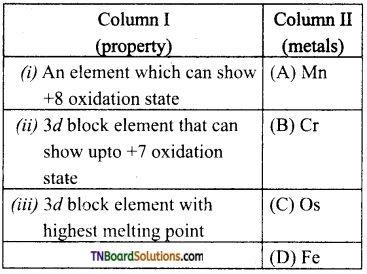(a) (i) – (C); (ii) – (A); (iii) – (B)
(b) (i) – (C); (ii) – (D); (in) – (A)
(c) (i) – (B); (ii) – (C); (iii) – (D)
(d) (i) – (D); (ii) – (C); (iii) – (A)
(a) (i) – (C); (ii) – (A); (iii) – (B)

12. Match statements given in column I with the oxidation states given in column II.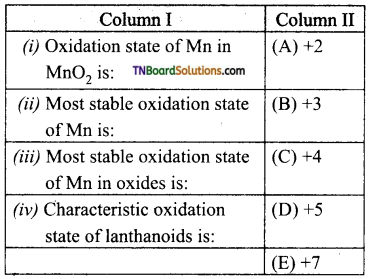(a) (i) – (C); (ii) – (A); (iii) – (E); (iv) – (B)
(b) (i) – (B); (ii) – (C); (iii) – (D); (iv) – (A)
(c) (i) – (D); (ii) – (B); (iii) – (C); (iv) – (A)
(d) (i) – (E); (ii) – (C); (iii) – (A); (iv) – (B)
(a) (i) – (C); (ii) – (A); (iii) – (E); (iv) – (B)

13. Knowing that the chemistry of lanthanoids
(Ln) is determined by its +3 oxidation state, which of the following statement is incorrect?
(a) The ionic size of Ln(III) decrease in general with increasing atomic number.
(b) Ln(III) compounds are generally colourless.
(c) Ln(III) hydroxides are mainly basic in character.
(d) Because of large size of Ln(III) ions the bonding in its compounds is predominantly ionic in character.
(b) Ln(III) compounds are generally colourless.
Hint: Ln(III) compounds are generally coloured due to partly filled f orbitals which permit f-f transition.

14. Which of the following statements regarding cerium (atomic no.58) is incorrect?
(a) The common oxidation states of cerium are +3 and +4.
(b) +3 oxidation state of Ce is more stable than +4.
(c) The +4 oxidation state of Ce is not known in solution.
(d) Cerium (IV) acts as an oxidising agent.
(c) The +4 oxidation state of Ce is not known in solution.15. MnO4 reacts with Br in alkaline pH to give:
(a) BrO3, MnO2
(b) Br2, MnO4-2
(c) Br2, MnO2
(d) BrO, MnO4-2
(a) BrO3, MnO2
Hint: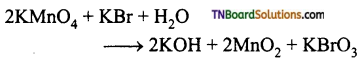16. Amount of oxalic acid present in a solution can be determined by titration with KMnO4 solution in the presence of H2SO4. The titration gives unsatisfactory result when carried out in the presence of HCl because HCl:
(a) reduces permanganate to Mn+2.
(b) oxidises oxalic acid to carbondioxide and water.
(c) gets oxidised by oxalic acid to chlorine.
(d) furnishes H+ ions in additional to those from oxalic acid.
(a) reduces permanganate to Mn+2.
Hint: In the presence of HCl, KMnO4 not only oxidises oxalic acid but also oxidises HCl to Cl2 and itself reduced to Mn+2.

17. The spin only magnetic moment of Fe+2 (in Bμ) is approximately:
(a) 4
(b) 1
(c) 5
(d) 6
(c) 5
Hint: Fe+2 = 3d6 4s0. It has 4 unpaired electron.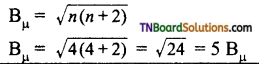18. Electronic configuration 6f a transition element X in +3 oxidation state is [Ar]3d5. What is its atomic number?
(a) 25
(b) 26
(c) 27
(d) 24
(b) 26
Hint: X+3 = [Ar]18 3d5 = 23 electrons As X+3 ion is formed by the loss of 3 electrons from X, X will have 26 electrons. Hence its at. no. = 26 V19. Metallic radii of some elements are given below: Which of these elements will have highest density?

 Element Fe Co Ni Cu Metallic radii/pm 126 125 125 128

(a) Fe
(b) Ni
(c) Co
(d) Cu
(d) Cu
Hint: $$\text { Density }=\frac{\text { mass }}{\text { volume }}$$
As we move from Fe to Cu mass increases and volume decreases. Hence, density increases. Increase in mass of Cu dominates over small increase in volume.

20. The magnetic nature of elements depends on the presence of unpaired electrons. Identify the configuration of transition element which shows highest magnetic moment:
(a) 3d7
(b) 3d5
(c) 3d8
(d) 3d2
(b) 3d5
Hint: 3d5 has maximum number of impaired electron.

21. There are 14 elements in actinoid series which of the following elements does not belong to this series?
(a) Cl
(b) Np
(c) Tm
(d) Fm
(c) Tm
Hint: Actinoid series is with atomic numbers 90 to 103. Thulium (Tm) has atomic number 69.

22. Gadolinium belongs to 4/series. Its atomic number is 64. Which of the following is the correct electronic configuration of gadolinium?
(a) [Xe] 4f7 5d1 6s2
(b) [Xe] 4f6 5d2 6s2
(c) [Xe] 4f8 6s2
(d) [Xe] 4f9 5s1
(a) [Xe] 4f7 5d1 6s2
Hint: [Xe]54 4f7 5d1 6s2 due to extra stability of half filled 4f sub shell.

23. Highest oxidation state of manganese in fluoride is +4(MnF4) but the highest oxidation state in oxides is +7(Mn2O7) because:
(a) fluorine is more electronegative than oxygen.
(b) fluorine does not possess ‘d’ orbital.
(c) fluorine stabilises lower oxidation state.
(d) in covalent compounds, fluorine can form only single bonds white oxygen can form double bonds.
(d) in covalent compounds, fluorine can form only single bonds white oxygen can form double bonds.

24. Assertion: Mercury is not considered as a transition element.
Reason: Mercury is a liquid.
(a) If both assertion and reason are true and the reason is the correct explanation of the assertion.
(b) If both assertion and reason are true but the reason is not the correct explanation of assertion.
(c) If an assertion is true, but the reason is false.
(d) If both assertion and reason are false.
(b) If both assertion and reason are true but the reason is not the correct explanation of assertion.
Hint: Mercury has completely filled ‘d’ orbitals.25. Assertion: in any transition series the magnetic moment of M+2 ions first increases and then decreases.
Reason: in a transition series, the number of unpaired electrons increases and then decreases.
(a) if both assertion and reason are true and the reason is the correct explanation of the assertion.
(b) if both assertion and reason are true but the reason is not the correct explanation of assertion.
(c) if the assertion is true, but the reason is false.
(d) if both assertion and reason are false.
(a) if both assertion and reason are true and reason is the correct explanation of the assertion.

26. Match the ions in list I with their corresponding property in list II.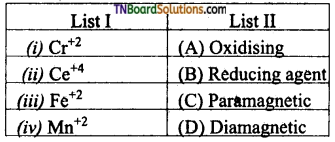(a) (i) – (B) & (C); (ii) – (A) & (D); (iii) – (B) & (C); (iv) – (C)
(b) (i) – (C); (ii) – (A) & (B); (iii) – (D); (iv) – (B) & (C)
(c) (i) – (B) & (C); (ii) – (B); (iii) – (A) & (D); (iv) – (B)
(d) (i) – (D); (ii) – (C); (iii) – (B); (iv) – (A), (D)
(a) (i) – (B) & (C); (ii) – (A) & (D); (iii) – (B) & (C); (iv) – (C)

27. The complex forming tendency of a transition metal depends upon:
(a) the availability of number of vacant ‘d’ orbitals.
(b) high ionisation energy.
(c) large size of the cation or high charge density.
(d) variable oxidation states.
(a) the availability of a number of vacant ‘d’ orbitals.

28. Which lanthanide is most commonly used?
(a) La
(b) No
(c) Th
(d) Ce
(a) La

29. In which of the following pairs are both the ions coloured in an aqueous solution?
(a) Ni+2, Ti+3
(b) Ni+2, Cu+
(c) Sc+3, CO+2
(d) Sc+3, Ti+3